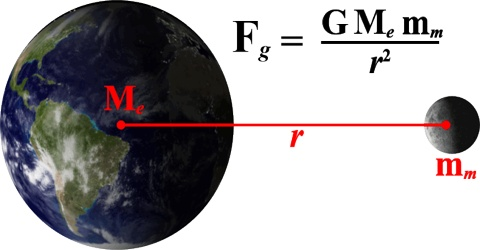Physics

# Newton’s Law of Gravitation in DynamicsNewton’s Law of Gravitation

The law of gravitation discovered in 1687 by the famous scientist Sir Isaac Newton by observing the fall of an apple and movement of planet and satellite is stated below:

Statement: Any two particles in the universe attract each other with a force which is directly proportional to the product of their masses, inversely proportional to the square of the distance between them and this force acts along the line joining the two particles or objects.Explanation: Let two particles of masses m1 and m2 be separated by a distance d [Figure] and let the force between them be F. Then according to Newton’s law of gravitation,

(i) F ∞ m1 m2 (when d is constant) and

(ii) F ∞ 1/r2 (when m1 and m2 are constants)

Combining (i) and (ii), we get,

F ∞ (m1m2/d2) [when m1, m2 and d vary]

or, F = Constant (m1m2/d2)

or, F = G (m1m2/d2)

Here, G is a constant of proportionality. This constant is known as Gravitational constant or Universal gravitational constant. G is called universal constant, because it does not depend on the nature of medium between the particles, nor does it depend on permeability, susceptibility, directivity and physical conditions of the particles.

S. I. unit of G is Newton and its dimension, [G] = M-1 T-2 L3

Newton’s gravitational constant is extremely small when expressed in terms of laboratory sized objects: the gravitational force between two 1 kg objects separated by 1 m is only 6.67 x 10-11 Newtons.